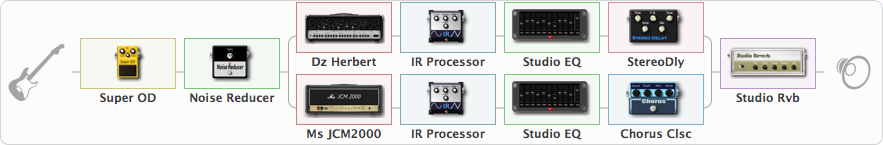# Def Lep PSSOME 1987

Discussion in 'ToneLib-GFX presets' started by Fergal Keogh, May 19, 2023.

1. Def Lep PSSOME 1987

Preset name: Def Lepard 80s Lead

Effects chain:Effect: "Super OD" (Overdrive / Distortion), active - "yes"
{
"Drive" = 39
"Tone" = 70
"Level" = 100
}

Effect: "Noise Reducer" (Dynamics / Filter), active - "yes"
{
"Sens" = 66
"Mode" = Hard
}

Effect: "Splitter" (Dynamics / Filter)
{
"A-Bypass" = Off
"A-Pan" = 0
"A-Level" = 55
"B-Bypass" = Off
"B-Pan" = 0
"B-Level" = 55
"Width" = 0

'A' branch:
{

Effect: "Dz Herbert" (Amp simulators), active - "yes"
{
"Gain" = 12
"Bass" = 65
"Middle" = 35
"Treble" = 80
"Presence" = 75
"Master" = 70
"Level (dB)" = 2
}

Effect: "IR Processor" (Cabinets), active - "yes"
{
"IR" = ACE EV 5153ST 1a
"Low Cut (Hz)" = 58
"Hi Cut (kHz)" = 20.0
"Mix" = 100
"Level (dB)" = 1
}

Effect: "Studio EQ" (Dynamics / Filter), active - "yes"
{
"31 Hz" = -2
"62 Hz" = -2
"125 Hz" = 4
"250 Hz" = -2
"500 Hz" = -4
"1 kHz" = 4
"2 kHz" = 4
"4 kHz" = 4
"8 kHz" = 4
"16 kHz" = 1
"Level (dB)" = 0
}

Effect: "StereoDly" (Delay), active - "yes"
{
"Time" = 756
"Feedback" = 44
"Tone" = 76
"Sens" = 0
"Mix" = 47
}
}
'B' branch:
{

Effect: "Ms JCM2000" (Amp simulators), active - "yes"
{
"Gain" = 13
"Bass" = 39
"Middle" = 62
"Treble" = 56
"Presence" = 85
"Master" = 70
"Level (dB)" = 2
}

Effect: "IR Processor" (Cabinets), active - "yes"
{
"IR" = ACE EV 5153ST 4b
"Low Cut (Hz)" = 41
"Hi Cut (kHz)" = 20.0
"Mix" = 100
"Level (dB)" = 2
}

Effect: "Studio EQ" (Dynamics / Filter), active - "yes"
{
"31 Hz" = -2
"62 Hz" = -2
"125 Hz" = 4
"250 Hz" = -2
"500 Hz" = -4
"1 kHz" = 4
"2 kHz" = 4
"4 kHz" = 4
"8 kHz" = 4
"16 kHz" = 1
"Level (dB)" = 0
}

Effect: "Chorus Clsc" (Modulation / Sfx), active - "yes"
{
"Speed" = 3.5
"Depth" = 58
"Center" = 5.5
"Mode" = Stereo
}
}
}

Effect: "Studio Rvb" (Reverberation), active - "yes"
{
"Time" = 7.3
"PreDelay" = 48
"LoDamp" = 21
"HiDamp" = 23
"Mix" = 30
}

Note: You will need to download and install the ToneLib-GFX software to use the preset.

File size:
15 KB
Views:
337